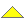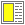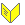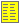Test of Object-oriented Programs - slide 17 : 35

Example of Equivalence Partitioning (2)
```  // Find the largest element in table in between the indexes from and to.
// Return the index of this element.
// Precondition: 0 <= from <= to <= table.Length-1
public int FindMaxIndex<T>(T[] table, int from, int to)
where T: IComparable<T> {
...
}   ```
• Equivalence partitions - all combinations of

• table

• table is empty/singular, largest element is first in table, largest element is last in table, largest element is in mid of table, all elements in table are equal

• from and to

• from and to are located at the boundaries of table

• from = to

• from = to - 1

• from and to are not close to each other